面试题:排列组合问题

年薪达不到25W大数据工程师、拿不到Offer全额退款->>>1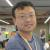function charsMap(o){
o = (o+"").replace(/\s+/g,"");
switch(o.length){
case 0:
case 1: return [o];
default:
var p = /^(\S+?)(\S)\$/.exec(o),
_r = charsMap(p),
l = p,
r = [];

for (var i = 0; i < _r.length; i++) {
var t = _r[i];
for (var j = 0, len = t.length; j <= len; j++) {
r.push( t.replace( new RegExp("^(\\S{"+j+"})(\\S{"+(len-j)+"})\$"), "\$1"+l+"\$2" ) );
}
}
return r;
}
}

var arr = "abcde";
var result = charsMap(arr).sort();

console.log( result );

0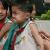abcde acdeb .....

bacde ......

c......

d...

e.....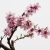0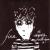def permutate(cur, l):
if cur:
print cur

for elem in l:
newL = [e for e in l if e != elem]
permutate(cur + elem, newL)

permutate('', ['a', 'b', 'c', 'd', 'e'])

0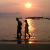public static void main(String[] args) {

String str = "abcde";

combi(str, "");

}

private static void combi(String str, String result) {

if (str.length() == 0) {
System.out.println(result);
return;
}

for (int i = 0; i < str.length(); i++) {
String str2 = str.substring(0, i) + str.substring(i + 1);
combi(str2, result + str.charAt(i));
}

}

0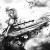public class GenerateString {

private String string;

public GenerateString(String letters) {
this.string = letters;
}

public void print() {
LinkedList<String> letters = spilitString();
exchange(letters, letters.size());
}

public void exchange(LinkedList<String> letters, int n) {
if (n > 2) {
exchange(letters, n - 1);
} else if (n == 2) {
getListString(letters);
}
for (int i = 0; i < n - 1; i++) {
for (int j = 0; j < n; j++) {
}
for (int j = 0; j < n; j++) {
}
if (n > 2) {
exchange(letters, n - 1);
} else if (n == 2) {
getListString(letters);
}
}
}

public void getListString(LinkedList<String> letters) {
for (int i = 0; i < letters.size(); i++) {
System.out.print(letters.get(i));
}
System.out.println();
}

public LinkedList<String> spilitString() {
for (int i = 0; i < string.length(); i++) {
letters.add(string.substring(i, i + 1));
}
return letters;
}

public static void main(String[] args) {
GenerateString g = new GenerateString("abcde");
g.print();
}
}

0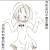0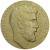programming erlang中变位词的例子
perms([]) -> [[]];
perms(L) -> [[H|T] || H<-L, T<-perms(L--[H])].